# 基于逆螺旋机构的电梯缓冲器设计及动力学特性分析Design of Elevator Buffer Based on Inverse Screw Mechanism and Dynamic Characteristics Analysis

DOI: 10.12677/MET.2019.84036, PDF, HTML, XML, 下载: 350  浏览: 540

Abstract: The buffer is the key device for ensuring passenger safety when an emergency occurs. In this paper, a new type of elevator buffer with constant-force is designed by using cam-spring constant torque and inverse screw drive mechanism. The working principle of the buffer is studied based on the impact dynamics theory, and the dynamic characteristics analysis and dynamic simulation, the performance curve of the buffer and the performance optimization parameters are obtained. The results show that the buffer has excellent cushioning performance, with the advantage of not taking up the pit space.

1. 引言

2. 新型电梯缓冲器方案设计

2.1. 恒力矩机构设计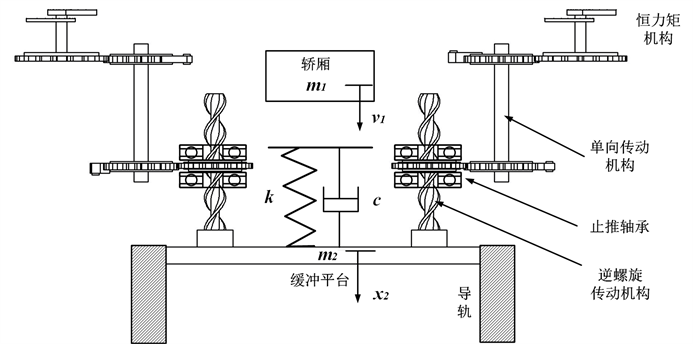Figure 1. Sketch of a new type of high speed elevator buffer

$k\left(f+\Delta {s}_{i}\right){r}_{i}=T$ (1)

f——弹簧初始伸长量，单位mm；

$\Delta {s}_{i}$ ——凸轮任意角度对应的弧长，单位mm；

${r}_{i}$ ——凸轮任意角度对应的半径，单位mm；

T——恒定力矩值，单位Nm。

$\frac{{r}_{i+1}+{r}_{i}}{2}\Delta {\theta }_{i}=\Delta {{s}^{\prime }}_{i}=\Delta {s}_{i+1}-\Delta {s}_{i}$ (2)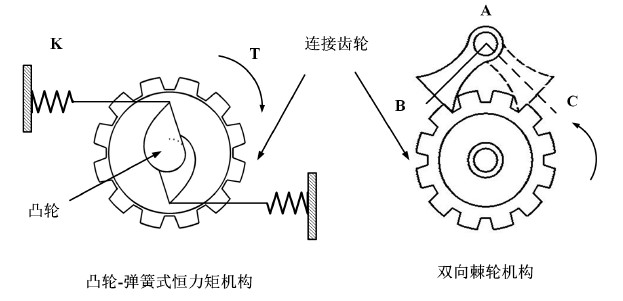Figure 2. Sketch of Constant torque mechanism and bidirectional

$\Delta {{s}^{\prime }}_{i}$ ——凸轮转过角度对应的弧长，mm。

$\Delta {s}_{1}=\frac{{r}_{2}-{r}_{1}}{{r}_{2}}f$ (3)

${r}_{1}$ ——凸轮初始半径，mm；

${r}_{2}$ ——凸轮转过 $\Delta \theta$ 后的半径，mm。

2.2. 逆螺旋传动机构设计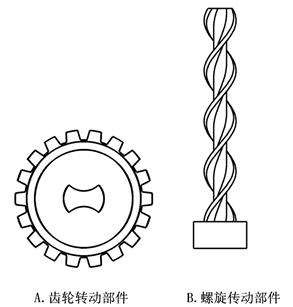Figure 3. Sketch of reverse screw drive mechanism

2.3. 新型缓冲器工作原理

3. 逆螺旋传动机构受力分析与仿真计算

3.1. 逆螺旋机构受力分析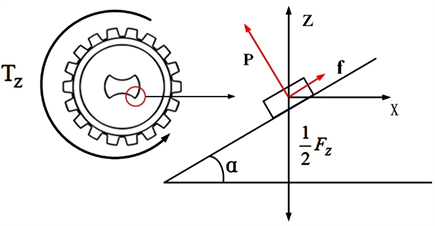Figure 4. Sketch of force analysis

$\left\{\begin{array}{l}{F}_{Z}={F}_{P}=2p\left(\mathrm{cos}\alpha +\mu \mathrm{sin}\alpha \right)\\ {T}_{Z}={T}_{P}=2r{F}_{X}=2rp\left(\mathrm{sin}\alpha -\mu \mathrm{cos}\alpha \right)\end{array}$ (4)

r——圆柱螺旋线半径，单位m；

μ——滑动摩擦系数；

p——碰撞接触力，单位N；

FP——接触力在Z方向的分力，单位N；

TP——接触力在Z方向的扭矩，单位N·m；

FX——接触力在X方向的分力，单位N；

FZ——Z方向上外力，单位N；

TZ——Z方向上外力矩，单位N·m。

$\lambda =\frac{{F}_{P}}{{T}_{P}}=\frac{{F}_{Z}}{{T}_{Z}}=\frac{1+\mu \mathrm{tan}\alpha }{r\left(\mathrm{tan}\alpha -\mu \right)}$ (5)

$x=\frac{h}{2\text{π}}\theta =\mathrm{tan}\alpha \cdot r\theta$ (6)

h——圆柱螺旋线导程，单位m。

$\stackrel{¨}{x}=\frac{h}{2\text{π}}\stackrel{¨}{\theta }=\mathrm{tan}\alpha \cdot r\stackrel{¨}{\theta }$ (7)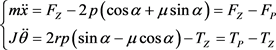(8)

J——转盘转动惯量，单位kg·m2

$\left\{\begin{array}{l}{F}_{Z}=m\stackrel{¨}{x}+\frac{2\pi J}{h}\lambda \stackrel{¨}{x}+\lambda {T}_{Z}\\ {F}_{P}=\frac{2\pi J}{h}\lambda \stackrel{¨}{x}+\lambda {T}_{Z}\end{array}$ (9)

3.2. 逆螺旋机构运动仿真分析Figure 5. Dynamic simulation model

$F=\left\{\begin{array}{l}0\text{}q>{q}_{0}\\ k{\left({q}_{0}-q\right)}^{e}-{c}_{\mathrm{max}}\cdot \left(\text{d}q/\text{d}t\right)\cdot step\left(q,{q}_{0}-d,1,{q}_{0},0\right)\text{}q\le {q}_{0}\end{array}$ (10)

q——碰撞过程中的实际距离；

k——刚度系数，单位穿透深度所产生的力；

e——碰撞过程中非线性刚度力指数；

${c}_{\mathrm{max}}$ ——接触边界的最大阻尼系数；

d——切入深度。

$step=\left\{\begin{array}{l}{h}_{0}\text{}x\le {x}_{0}\\ {h}_{0}+a\cdot {\Delta }^{2}\left(3-2\Delta \right)\text{}{x}_{0} (11)

$\left\{\begin{array}{l}\stackrel{˙}{x}=\frac{h}{2\pi }\stackrel{˙}{\theta }=\mathrm{tan}\alpha \cdot r\stackrel{˙}{\theta }\\ \stackrel{¨}{x}=\frac{h}{2\pi }\stackrel{¨}{\theta }=\mathrm{tan}\alpha \cdot r\stackrel{¨}{\theta }\end{array}$ (12)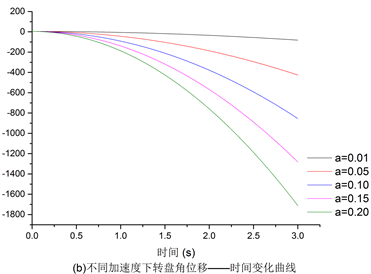Figure 6. Results of dynamic simulationTable 1. Error analysis of angular velocity simulationTable 2. Error analysis of angular acceleration simulation

3.3. 逆螺旋机构碰撞接触力仿真分析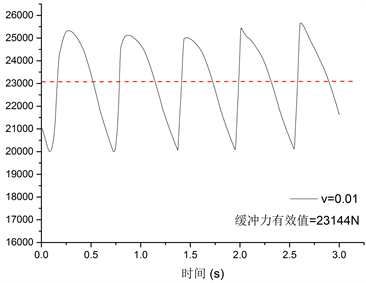(a) v = 0.01 m/s时缓冲力–时间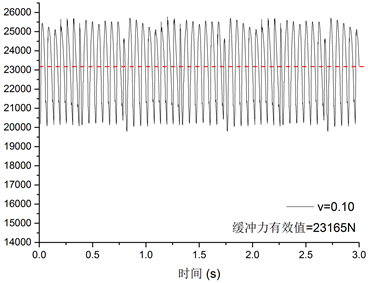(b) v = 0.10 m/s时缓冲力–时间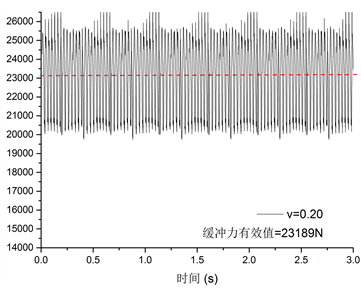(c) v = 0.20 m/s时缓冲力–时间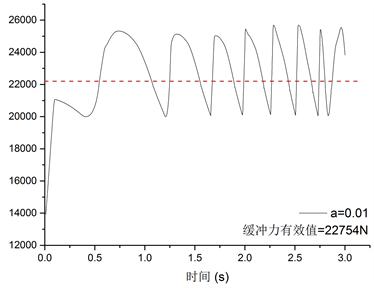(d) a = 0.01m/s2时缓冲力–时间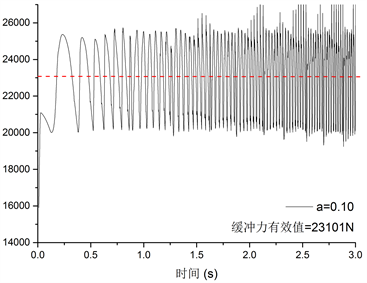(e) a = 0.10 m/s2时缓冲力–时间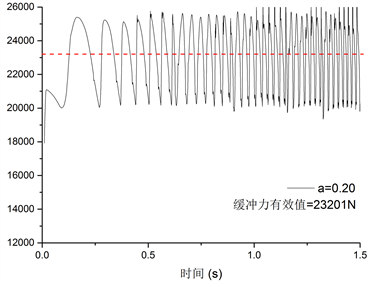(f) a = 0.20 m/s2时缓冲力–时间

Figure 7. The results of dynamic simulationTable 3. Error analysis of buffer force simulation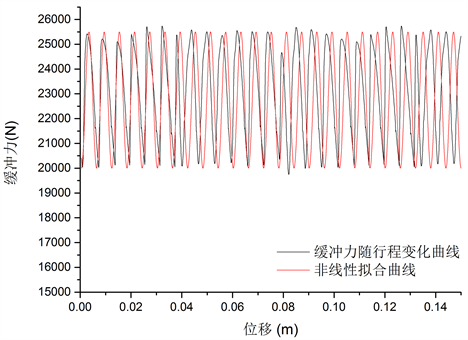Figure 8. Buffer force versus displacement curve

$y={y}_{0}+A\ast \mathrm{sin}\left[\frac{\pi }{\omega }\left(x-{x}_{c}\right)\right]$ (13)

$F=23140+2400\ast \mathrm{sin}\left[\frac{\pi }{0.003}\left(x+0.004\right)\right]$ (14)

4. 冲击响应求解与数值仿真计算

4.1. 冲击响应求解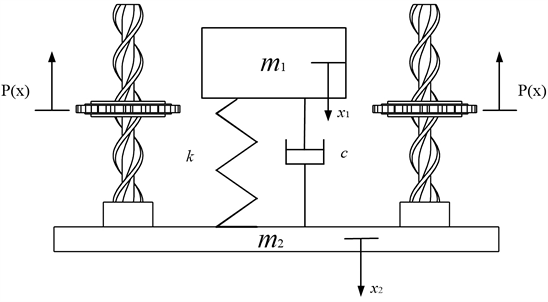Figure 9. Dynamic model of buffer

$\left\{\begin{array}{l}{m}_{1}{\stackrel{¨}{x}}_{1}={m}_{1}g-k\left({x}_{1}-{x}_{2}\right)-c\left({\stackrel{˙}{x}}_{1}-{\stackrel{˙}{x}}_{2}\right)\\ {m}_{2}{\stackrel{¨}{x}}_{2}={m}_{2}g+k\left({x}_{1}-{x}_{2}\right)+c\left({\stackrel{˙}{x}}_{1}-{\stackrel{˙}{x}}_{2}\right)-nP\left(x\right)\end{array}$ (15)

${m}_{2}$ ——缓冲器缓冲平台质量，单位kg；

${x}_{1}$ ——电梯轿厢位移，单位m；

${x}_{2}$ ——缓冲平台位移，单位m；

k——弹簧刚度系数，单位N/m；

c——阻尼系数，单位N·s/m；

n——逆螺旋装置个数。

$P\left(x\right)=\left\{\begin{array}{l}{F}_{Z}\text{}\text{\hspace{0.17em}}\text{\hspace{0.17em}}{F}_{Z}\le {F}_{P}&{\stackrel{˙}{x}}_{2}=0\\ \frac{2\pi J}{h}\lambda {\stackrel{¨}{x}}_{2}+P\text{}\text{\hspace{0.17em}}\text{\hspace{0.17em}}{F}_{Z}>{F}_{P}\text{\hspace{0.17em}}\text{or}\text{\hspace{0.17em}}{\stackrel{˙}{x}}_{2}>0\end{array}$ (16)

FP——碰撞接触力在Z方向的分力，单位N；

P——缓冲力有效值，单位N。

$n{F}_{Z}={m}_{2}g+k\left({x}_{1}-{x}_{2}\right)+c\left({\stackrel{˙}{x}}_{1}-{\stackrel{˙}{x}}_{2}\right)$

1) 缓冲初始阶段，在恒力矩机构和单向传动机构的作用下，缓冲平台保持静止直到Z方向外力达到临界值P；

2) 在缓冲运动过程中缓冲力P(x)与加速度有关，相当于增加了缓冲平台的等效质量；

$x={x}_{1}-{x}_{2}$ 整理得到相对运动微分方程：

$\stackrel{¨}{x}+\frac{c}{m}\stackrel{˙}{x}+\frac{k}{m}x={P}^{\prime }$ (17)

$\left\{\begin{array}{l}m=\frac{{m}_{1}\left({m}_{2}+\frac{2\pi nJ}{h}\lambda \right)}{{m}_{1}+{m}_{2}+\frac{2\pi nJ}{h}\lambda }\\ {P}^{\prime }=\frac{nP+\frac{2\pi nJ}{h}\lambda g}{{m}_{2}+\frac{2\pi nJ}{h}\lambda }\end{array}$

$x={\text{e}}^{-{\xi }^{\prime }\omega t}\left[A\mathrm{cos}\left({\omega }_{d}t\right)+B\mathrm{sin}\left({\omega }_{d}t\right)\right]+\frac{{P}^{\prime }m}{k}$ (18)

$\left\{\begin{array}{l}A={x}_{0}-\frac{{P}^{\prime }m}{k}\\ B=\frac{{v}_{0}+{\xi }^{\prime }\omega \left({x}_{0}-\frac{{P}^{\prime }m}{k}\right)}{{\omega }_{d}}\end{array}$ (19)

$\left\{\begin{array}{l}{\stackrel{¨}{x}}_{1}=g-\frac{{P}^{\prime }m}{{m}_{1}}-\frac{{\text{e}}^{-{\xi }^{\prime }\omega t}}{{m}_{1}}C\mathrm{sin}\left({\omega }_{d}t+\phi \right)\\ {\stackrel{¨}{x}}_{2}=\frac{{m}_{2}g+{P}^{\prime }m-nP}{{m}_{2}+\frac{2n\pi J}{h}\lambda }+\frac{{\text{e}}^{-{\xi }^{\prime }\omega t}}{{m}_{2}+\frac{2n\pi J}{h}\lambda }C\mathrm{sin}\left({\omega }_{d}t+\phi \right)\end{array}$ (20)

$\left\{\begin{array}{l}C={\left\{{\left[kA+c\left(B{\omega }_{d}-{\xi }^{\prime }\omega A\right)\right]}^{2}+{\left[kB-c\left(A{\omega }_{d}+{\xi }^{\prime }\omega B\right)\right]}^{2}\right\}}^{-\frac{1}{2}}\\ \phi =\mathrm{arctan}\left(\frac{kA+c\left(B{\omega }_{d}-{\xi }^{\prime }\omega A\right)}{kB-c\left(A{\omega }_{d}+{\xi }^{\prime }\omega B\right)}\right)\end{array}$

1) m1加速度由两个常数项和一个振幅不断衰减的波动项组成，符合匀减速缓冲的设计目标；

2) 轿厢缓冲过程的平均减速度主要由缓冲力有效值P决定；

3) 在弹簧刚度一定的情况下，轿厢最大减速度随着阻尼系数的增加先减小后增加；在阻尼系数一定的情况下，最大减速度随着刚度的增加而增加。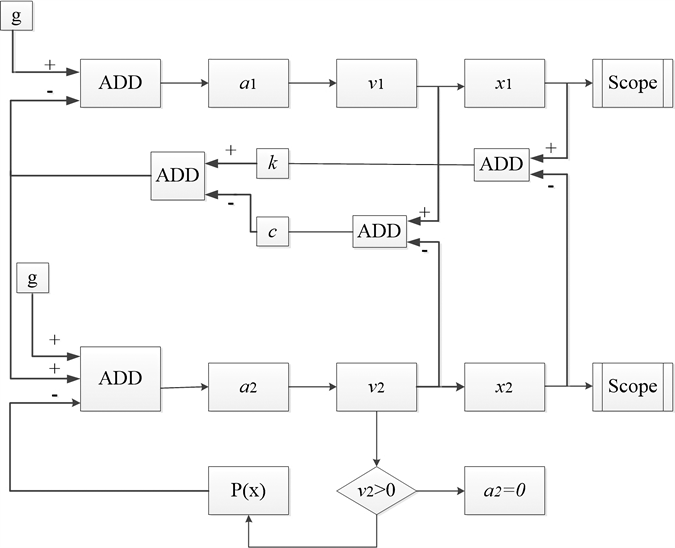Figure 10. Simulation block diagram

$F=12750+1800\ast \mathrm{sin}\left[\frac{\pi }{0.003}\left(x-0.0012\right)\right]$ (21)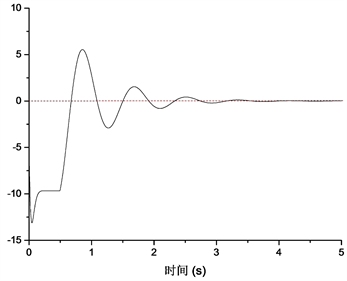(a) P(X)为有效值时冲击加速度–时间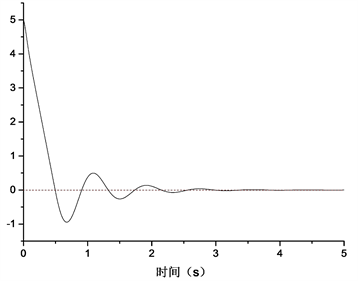(b) P(X)为有效值时冲击速度–时间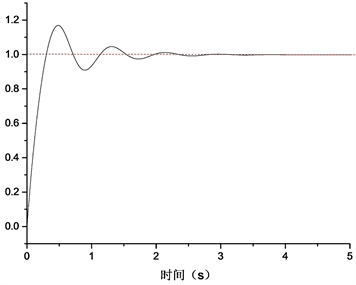(c) P(X)为有效值时冲击位移–时间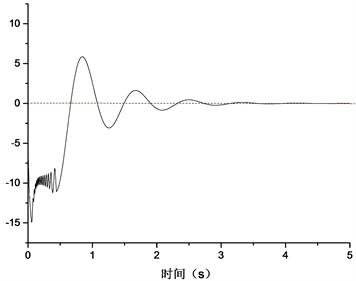(d) P(X)为拟合值时冲击加速度–时间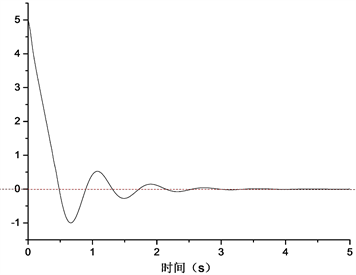(e) P(X)为拟合值时冲击速度–时间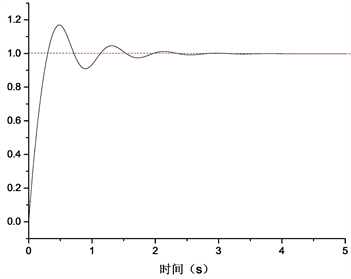(f) P(X)为拟合值时冲击位移–时间

Figure 11. Numerical results of impact response under different buffer force

$\left\{\begin{array}{l}\xi =\frac{c}{2\sqrt{{m}_{1}k}}\\ {H}_{C}=\frac{{a}_{\mathrm{max}}{x}_{\mathrm{max}}}{v}\end{array}$ (22)

xmax——m1缓冲过程中最大位移，单位m。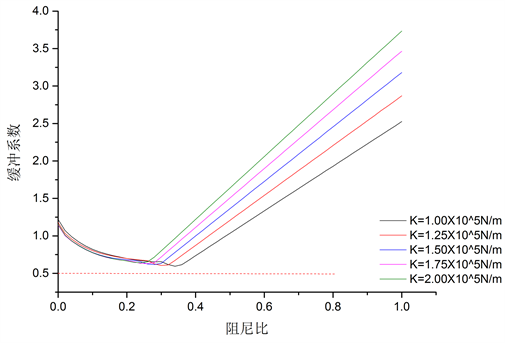Figure 12. The curves of buffer coefficient versus damping ratio under different stiffness coefficientsFigure 13. The curves of rebound velocity versus damping ratio under different stiffness coefficients

1) 缓冲系数随着阻尼比的增加，先减小后增大，存在最优阻尼比使得该刚度下的缓冲系数取得最小值；

2) 反弹速度随着阻尼比的增加，先增大后减小，且随着刚度的增加，反弹速度不断减小；

3) 如表4所示，随着刚度的增加，最优阻尼比与反弹速度不断减小，但最小缓冲系数不断增大。Table 4. Optimal parameters under different stiffness

5. 结语

NOTES

*通讯作者。

  GB7588-2003. 电梯制造与安装安全规范[S].  黄旭, 王德山. 试论侧压式电梯底坑缓冲装置设计[J]. 中国设备工程, 2018(2): 132-133.  姜青松. 电梯安全部件试验系统动力学及试验研究[D]: [硕士学位论文]. 武汉: 武汉轻工大学, 2016.  张利平. 缓冲器原理与应用[J]. 现代机械, 1999(1): 49-51.  陈占清. 活塞复位式液压缓冲器的研究[D]: [硕士学位论文]. 上海: 上海交通大学, 2007.  姚荣康, 朱昌明, 詹永麒, 周琼. 带气囊式蓄能器的油压缓冲器的冲击研究[J], 振动与冲击, 2006, 25(1): 153-155.  马培忠. 电梯用油压缓冲器原理与性能分析[J]. 中国电梯, 1995(2): 6-8.  王敏, 朱昌明, 詹永麒, 姚荣康. 一种带气囊式蓄能器的新型缓冲器的动态特性研究[J]. 机械设计与研究, 2004, 20(3): 84-85.  冯维明, 李辉. 恒力弹簧支吊架凸轮曲线方程推导[J]. 现代制造工程. 2006(8): 95-97.  Riley, R.Q. and Carey, D.L. (1979) Exercise Machine with Spring-Cam Arrangement for Equalizing the Force Required through the Exercise Stroke. US4231568, 1979-01-29.  陈建, 邓利娟. 恒力弹性机构的设计[J]. 中国机械工程, 2010, 21(16): 1944-1946.  Yan, H., Li, L.M., Hu, C.H., et al. (2011) Astronaut Mass Measurement Using Linear Acceleration Method and the Effect of Body Non-Rigidity. Science China: Physics, Mechanics & Astronomy, 54, 777-782. https://doi.org/10.1007/s11433-011-4296-y  Fujii, Y., Fujimoto, H. and Namioka, S. (1999) Mass Measurement under Weight-Less Conditions. Review of Scientific Instruments, 70, 111-113. https://doi.org/10.1063/1.1149550  Shimada, K. and Fujii, Y. (2010) Issues with Body Mass Measurement on the International Space Station (ISS). Applied Mechanics and Materials, 36, 9-20. https://doi.org/10.4028/www.scientific.net/AMM.36.9  Hou, C.W. and Lan, C.C. (2013) Functional Joint Mechanisms with Constant-Torque Outputs. Mechanism and Machine Theory, 62, 166-181. https://doi.org/10.1016/j.mechmachtheory.2012.12.002  牛福亮, 高海波, 刘振, 等. 一种串联张紧式恒力矩机构设计及实验研究[J]. 机器人, 2016, 38(4): 475-485.  梁锡昌, 蒋建东, 李润方, 陈小安. 特种螺旋传动机构的研究[J]. 机械工程学报, 2003, 39(10): 106-110.  梁锡昌, 王光建, 郑小光. 基于螺旋机构的旋转作动器研究[J]. 航空学报, 2003, 24(3): 282-285.  王光建. 基于逆滚动螺旋的机器人关节的研究[D]: [博士学位论文]. 重庆: 重庆大学, 2005.  翁雪涛, 朱石坚, 何琳. 限位器抗冲击计算[J]. 中国造船, 2002, 43(2): 85-89.